Question

# Given a standardized normal distribution with mean - 0 and standard deviation 1: d. What is the...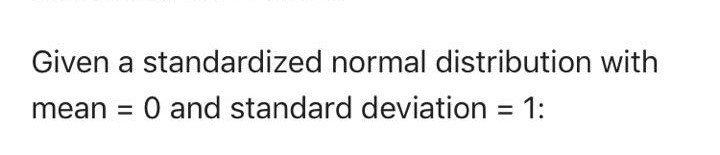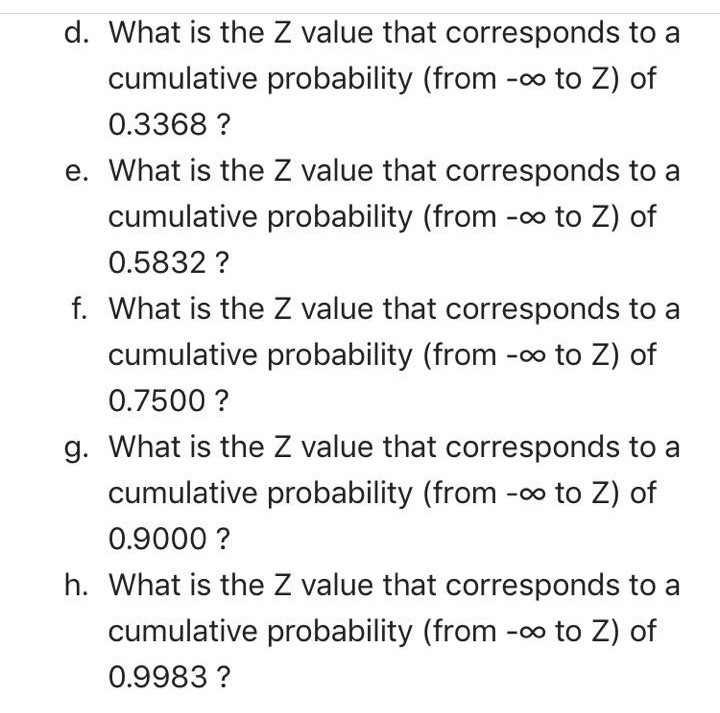Given a standardized normal distribution with mean - 0 and standard deviation 1:
d. What is the Z value that corresponds to a cumulative probability (from -oo to Z) of 0.3368? e. What is the Z value that corresponds to a cumulative probability (from -oo to Z) of 0.5832? f. What is the Z value that corresponds to a cumulative probability (from -oo to Z) of 0.7500? g. What is the Z value that corresponds to a cumulative probability (from -oo to Z) of 0.9000? h. What is the Z value that corresponds to a cumulative probability (from -oo to Z) of 0.9983?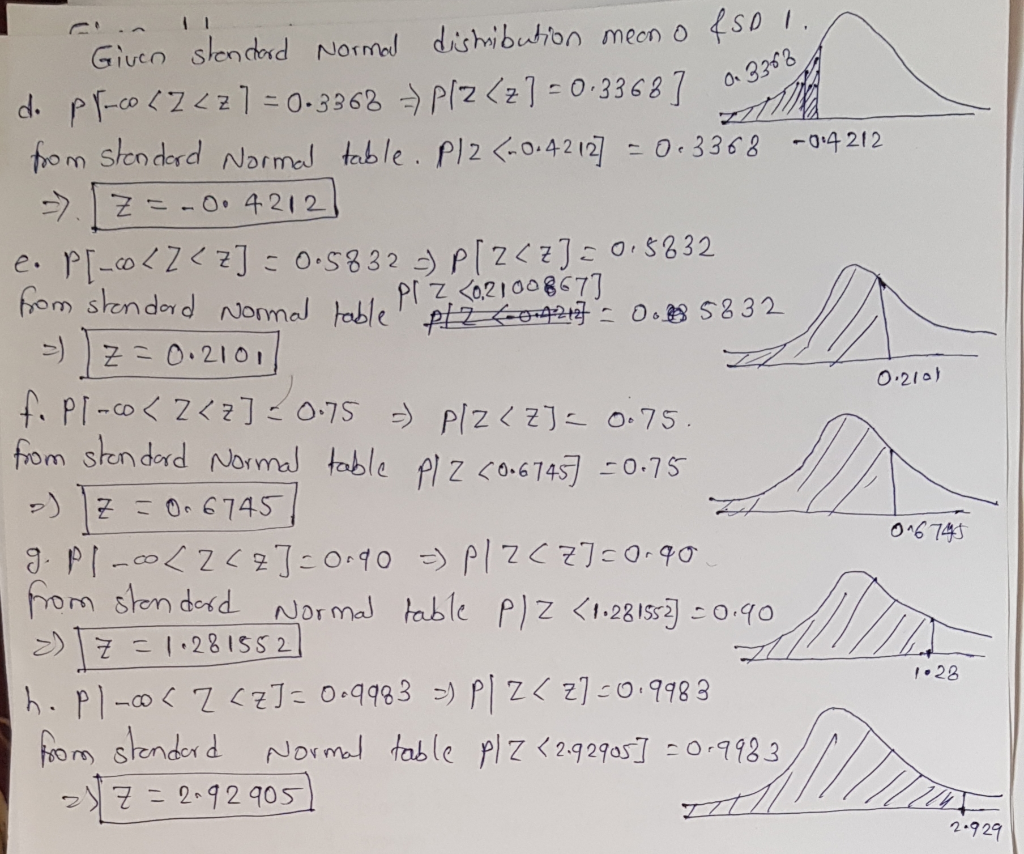#### Earn Coins

Coins can be redeemed for fabulous gifts.

Similar Homework Help Questions
• ### Given a standardized normal distribution (with a mean of 0 and a standard deviation of 1)...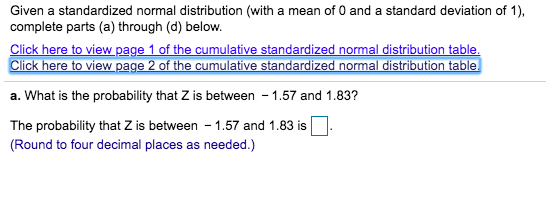Given a standardized normal distribution (with a mean of 0 and a standard deviation of 1) complete parts (a) through (d) below. Click here to view page 1 of the cumulative standardized normal distribution table, Click here to view page 2 of the cumulative standardized normal distribution table. a. What is the probability that Z is between 1.57 and 1.83? - The probability that Z is between 1.57 and 1.83 is (Round to four decimal places as needed.) particular train...

• ### Given a standardized normal distribution (with a mean of O and a standard deviation of 1),...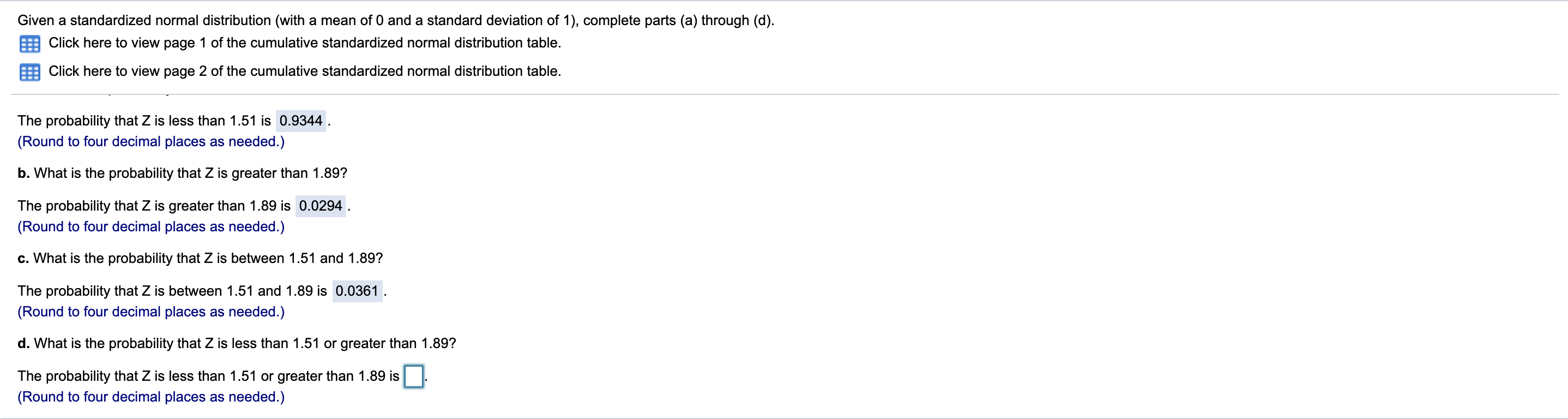Given a standardized normal distribution (with a mean of O and a standard deviation of 1), complete parts (a) through (d). 5 Click here to view page 1 of the cumulative standardized normal distribution table. E: Click here to view page 2 of the cumulative standardized normal distribution table. The probability that Z is less than 1.51 is 0.9344. (Round to four decimal places as needed.) b. What is the probability that Z is greater than 1.89? The probability that...

• ### Given a standardized normal distribution (with a mean of O and a standard deviation of 1),...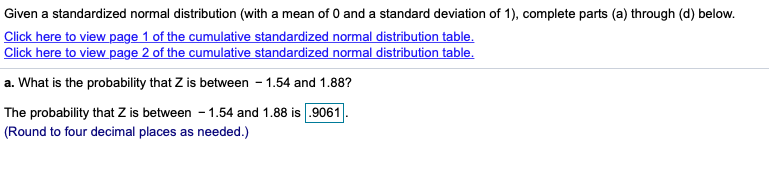Given a standardized normal distribution (with a mean of O and a standard deviation of 1), complete parts (a) through (d) below. Click here to view page 1 of the cumulative standardized normal distribution table Click here to view page 2 of the cumulative standardized normal distribution table. a. What is the probability that Z is between - 1.54 and 1.88? The probability that Z is between - 1.54 and 1.88 is .9061. (Round to four decimal places as needed.)

• ### Given a standardized normal distribution (with a mean of 0 and a standard deviation of 1,...

Given a standardized normal distribution (with a mean of 0 and a standard deviation of 1, as in Table E.2), what is the value of A that would satisfy, P(Z < A) = 0.102

• ### Given a standardized normal distribution (with a mean of O and a standard deviation of 1),...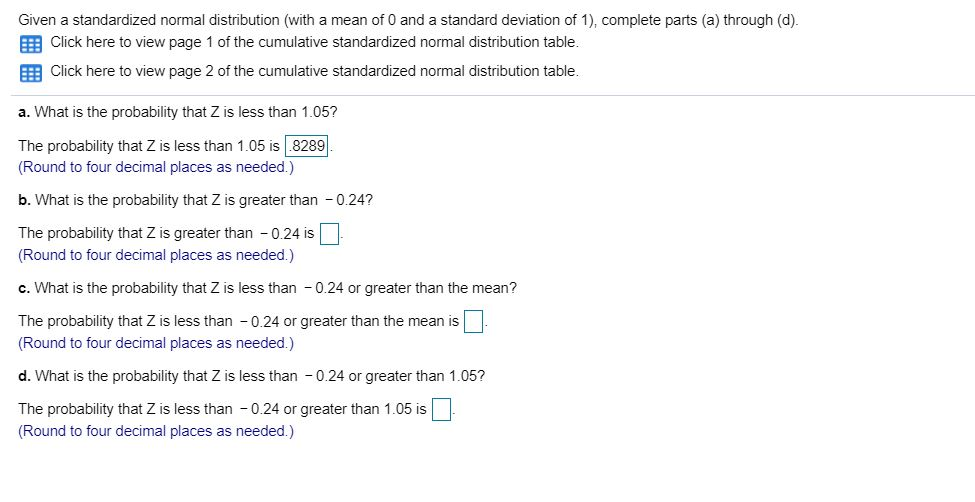Given a standardized normal distribution (with a mean of O and a standard deviation of 1), complete parts (a) through (d). Click here to view page 1 of the cumulative standardized normal distribution table. Click here to view page 2 of the cumulative standardized normal distribution table. a. What is the probability that Z is less than 1.05? The probability that Z is less than 1.05 is 8289 (Round to four decimal places as needed.) b. What is the probability...

• ### 6. A normal distribution of has a mean of 20 and a standard deviation of 10....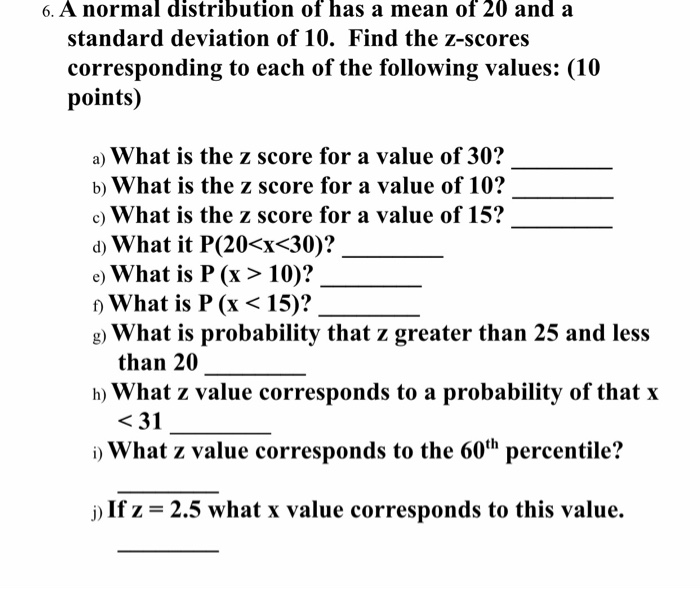6. A normal distribution of has a mean of 20 and a standard deviation of 10. Find the z-scores corresponding to each of the following values: (10 points) a) What is the z score for a value of 30? b) What is the z score for a value of 10? c) What is the z score for a value of 15? d) What it P(20<x<30)? e) What is P (x > 10)? ) What is P (x < 15)? g)...

• ### If X has a normal distribution with mean μ and standard deviation σ, and Z is...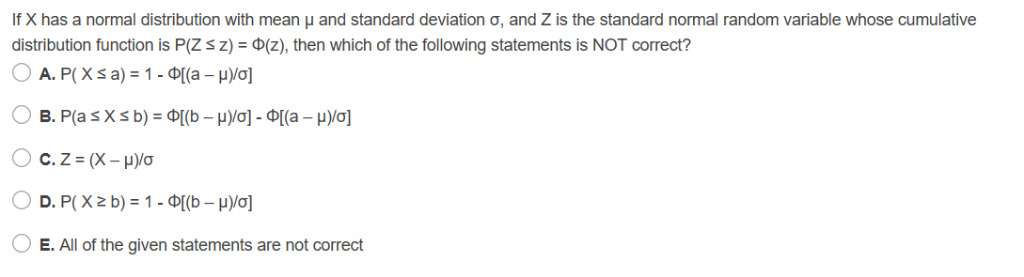If X has a normal distribution with mean μ and standard deviation σ, and Z is the standard normal random variable whose cumulative distribution function is P(Z s Z)-0(Z), then which of the following statements is NOT correct? O E. All of the given statements are not correct

• ### part B Given a sta Click here to view page 1 of the cumulative standardized normal...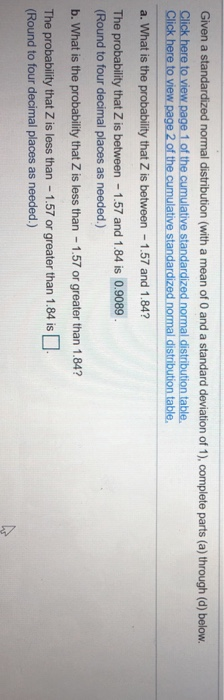part B Given a sta Click here to view page 1 of the cumulative standardized normal distribution table. Cick here to wew pege 2 of the cumulative standardized normal distibution table a. What is the probability that Z is between 1.57 and 1.84? The probability that Z is between 1.57 and 1.84 is 0.9089 (Round to four decimal places as needed.) b. What is the probability that Z is less than 1.57 or greater than 1.84? The probability that Z...

• ### Consider a normal distribution with mean 25 and standard deviation 5. What is the probability a...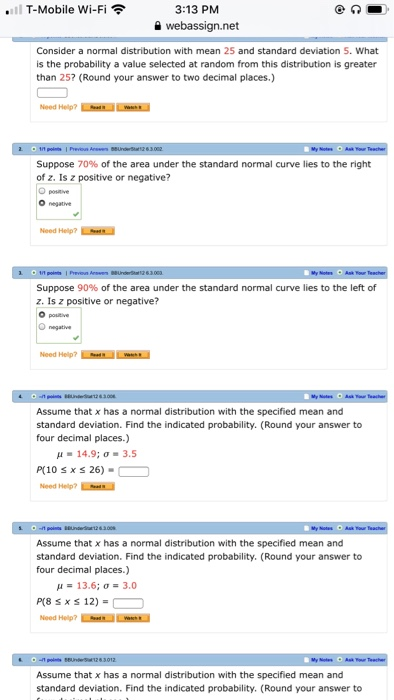Consider a normal distribution with mean 25 and standard deviation 5. What is the probability a value selected at random from this distribution is greater than 25? (Round your answer to two decimal places.) Assume that x has a normal distribution with the specified mean and standard deviation. Find the indicated probability. (Round your answer to four decimal places.) μ = 14.9; σ = 3.5 P(10 ≤ x ≤ 26) = Need Help? Read It Assume that x has a...

• ### 1. Assuming the Standard Normal Distribution, USING EXCEL find:          a. What is the probability of...

1. Assuming the Standard Normal Distribution, USING EXCEL find:          a. What is the probability of Z < than -1.75?           b. What is the probability of Z > than 1.00?           c. What is the probability of Z between 1.00 and 2.00?          d. 15% of the cumulative probability is above what value for Z?           e. 95% of the cumulative probability is below what value for Z?           f. What is the probability of Z<-2.00 OR X> 2.00?          ...